# 利用 PFC 电路减少谐波失真## 交流电的功率因数

$$\lambda = P / S$$

$$S = \sqrt{P^2 + Q^2}$$

PF 用于衡量有多少电力被有效利用。PF 值越大，表示其电力的利用率越高。

$$\lambda (PF) = \cos \varphi \times \frac {1}{\sqrt{1+\mathit{THD^2}}}$$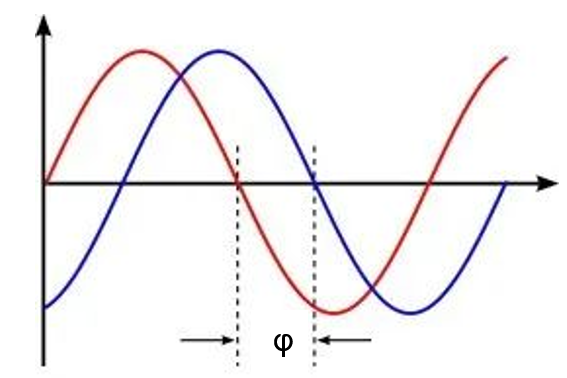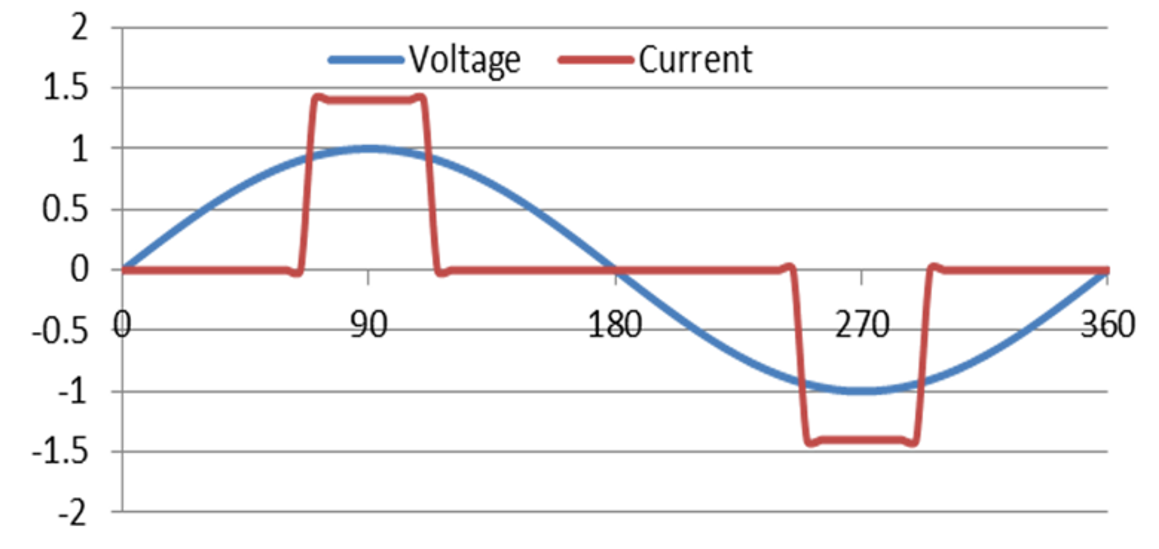$$Distortion : \frac {1}{\sqrt{1+\mathit{THD^2}}}$$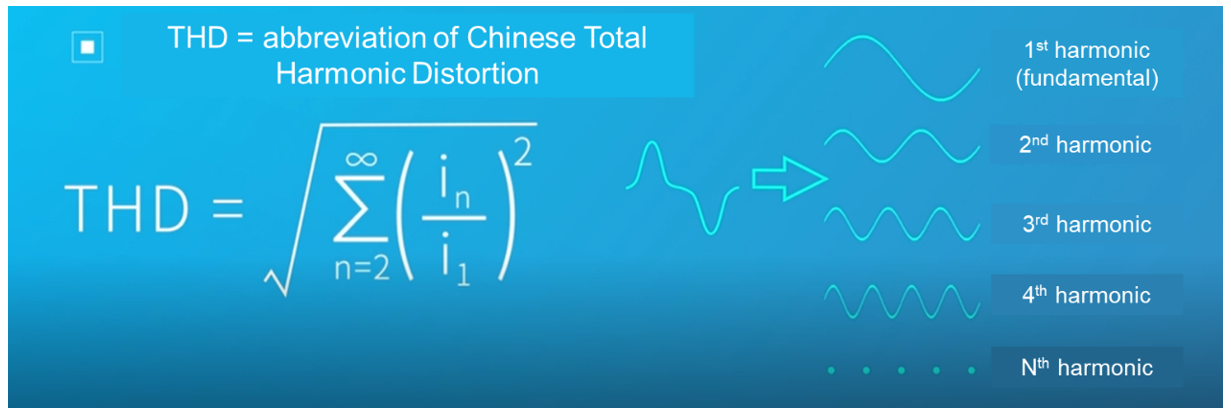## 利用电路来实现高功率因数校正 (PFC)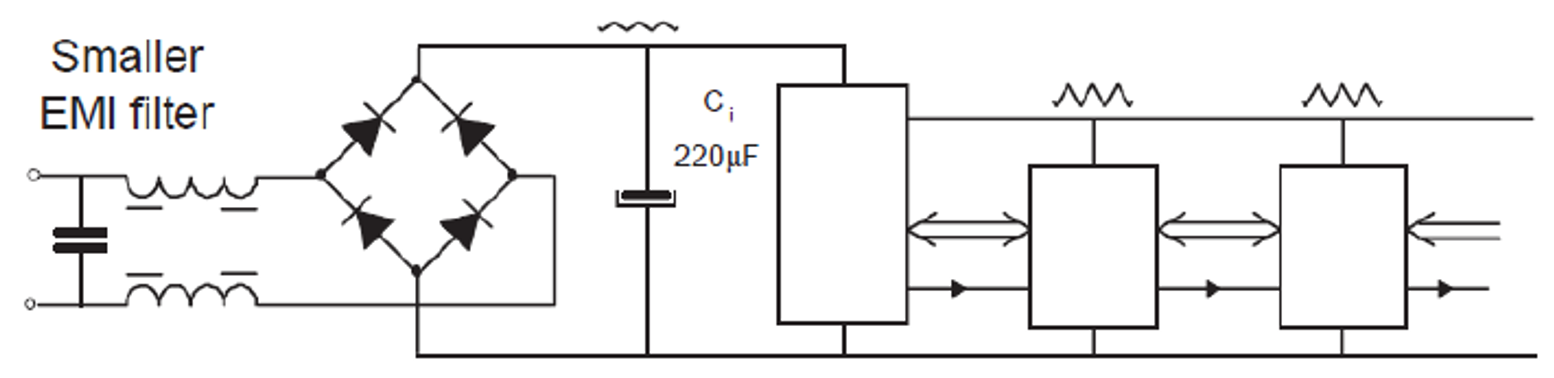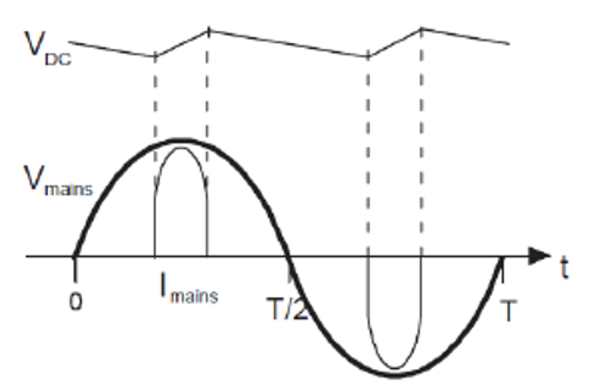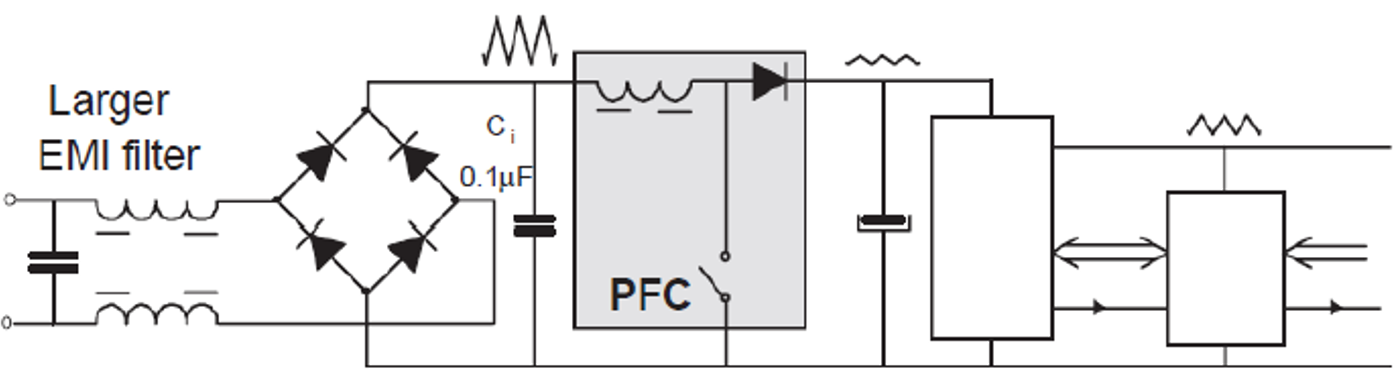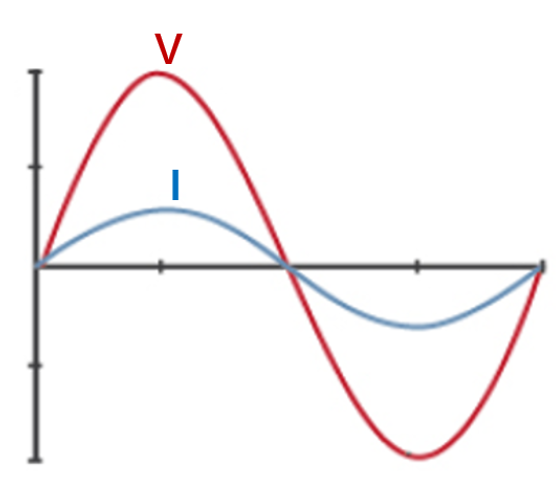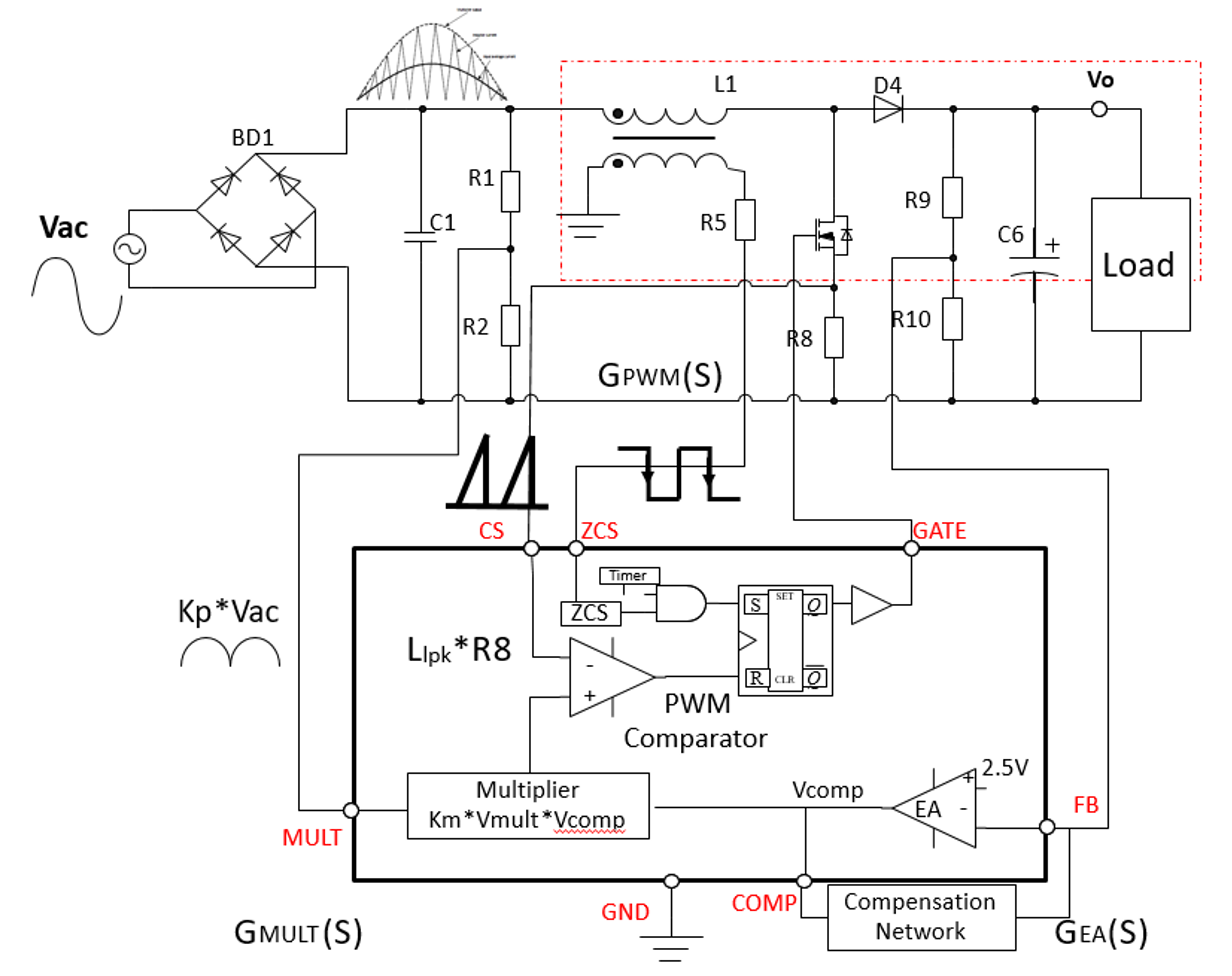APFC 电路采用典型的临界电流控制模式，这种控制模式在 300W 以内的电源设计中很常见。对更大功率的应用而言，则需选择连续导通模式 (CCM) PFC 电路。当对轻载效率有要求时，增加非连续导通模式（DCM）将有效降低工作频率，以此改善开关损耗与EMI。

## 结语

MPS 提供的一系列 PFC 控制器产品可以满足谐波电流的要求并提高电源质量。这些 PFC 控制器均采用专有封装技术和高效率的集成设计。